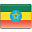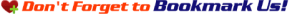Main Menu: Vocabulary Phrases Grammar Learn Amharic Flashcards Alphabet Quiz# Amharic Letters

Let's now learn the Amharic letters. Knowing these characters will allow you to read and write commonly used expressions and words with ease.

 ሐ [hä] as in hä ኀ [hä] as in hä ሃ [ha] as in ha ሓ [ha] as in ha ኃ [ha] as in ha ሁ [hu] as in hu ሑ [hu] as in hu ኁ [hu] as in hu ሂ [hi] as in hi ሒ [hi] as in hi ኂ [hi] as in hi ሄ [he] as in he ሔ [he] as in he ኄ [he] as in he ህ [hə] as in hə ሕ [hə] as in hə ኅ [hə] as in hə ሆ [ho] as in ho ሖ [ho] as in ho ኆ [ho] as in ho ለ [lä] as in lä ሉ [lu] as in lu ሊ [li] as in li ላ [la] as in la ሌ [le] as in le ል [lə] as in lə ሎ [lo] as in lo ሏ [wa] as in wa መ [mä] as in mä ሙ [mu] as in mu ሚ [mi] as in mi ማ [ma] as in ma ሜ [me] as in me ም [mə] as in mə ሞ [mo] as in mo ሟ [ma] as in ma ሠ [sä] as in sä ሰ [sä] as in sä ሡ [su] as in su ሱ [su] as in su ሢ [si] as in si ሲ [si] as in si ሣ [sa] as in sa ሳ [sa] as in sa ሤ [se] as in se ሴ [se] as in se ሥ [sə] as in sə ስ [sə] as in sə ሦ [so] as in so ሶ [so] as in so ረ [rä] as in rä ሩ [ru] as in ru ሪ [ri] as in ri ራ [ra] as in ra ሬ [re] as in re ር [rə] as in rə ሮ [ro] as in ro ሸ [šä] as in šä ሹ [šu] as in šu ሺ [ši] as in ši ሻ [ša] as in ša ሼ [še] as in še ሽ [šə] as in šə ሾ [šo] as in šo ቀ [qä] as in qä ቁ [qu] as in qu ቂ [qi] as in qi ቃ [qa] as in qa ቄ [qe] as in qe ቅ [qə] as in qə ቆ [qo] as in qo ቋ [qwa] as in qwa በ [bä] as in bä ቡ [bu] as in bu ቢ [bi] as in bi ባ [ba] as in ba ቤ [be] as in be ብ [bə] as in bə ቦ [bo] as in bo ተ [tä] as in tä ቱ [tu] as in tu ቲ [ti] as in ti ታ [ta] as in ta ቴ [te] as in te ት [tə] as in tə ቶ [to] as in to ቷ [ta] as in ta ቸ [čä] as in čä ቹ [ču] as in ču ቺ [či] as in či ቻ [ča] as in ča ቼ [če] as in če ች [čə] as in čə ቾ [čo] as in čo ነ [nä] as in nä ኑ [nu] as in nu ኒ [ni] as in ni ና [na] as in na ኔ [ne] as in ne ን [nə] as in nə ኖ [no] as in no ኘ [ñä] as in ñä ኙ [ñu] as in ñu ኚ [ñi] as in ñi ኛ [ña] as in ña ኜ [ñe] as in ñe ኝ [ñə] as in ñə ኞ [ño] as in ño አ [ʾä] as in ʾä ዐ [ʾä] as in ʾä ኡ [ʾu] as in ʾu ዑ [ʾu] as in ʾu ኢ [ʾi] as in ʾi ዒ [ʾi] as in ʾi ኣ [ʾa] as in ʾa ዓ [ʾa] as in ʾa ኤ [ʾe] as in ʾe ዔ [ʾe] as in ʾe እ [ʾə] as in ʾə ዕ [ʾə] as in ʾə ኦ [ʾo] as in ʾo ዖ [ʾo] as in ʾo ከ [kä] as in kä ኩ [ku] as in ku ኪ [ki] as in ki ካ [ka] as in ka ኬ [ke] as in ke ክ [kə] as in kə ኮ [ko] as in ko ኸ [hä] as in hä ኹ [hu] as in hu ኺ [hi] as in hi ኻ [ha] as in ha ኼ [he] as in he ኽ [hə] as in hə ኾ [ho] as in ho ወ [wä] as in wä ዊ [wi] as in wi ዋ [wa] as in wa ዌ [we] as in we ው [wə] as in wə ዉ [wu] as in wu ዎ [wo] as in wo ዘ [zä] as in zä ዙ [zu] as in zu ዚ [zi] as in zi ዛ [za] as in za ዜ [ze] as in ze ዝ [zə] as in zə ዞ [zo] as in zo ዠ [žä] as in žä ዡ [žu] as in žu ዢ [ži] as in ži ዣ [ža] as in ža ዤ [že] as in že ዥ [žə] as in žə ዦ [žo] as in žo የ [yä] as in yä ዩ [yu] as in yu ዪ [yi] as in yi ያ [ya] as in ya ዬ [ye] as in ye ይ [yə] as in yə ዮ [yo] as in yo ደ [dä] as in dä ዱ [du] as in du ዲ [di] as in di ዳ [da] as in da ዴ [de] as in de ድ [də] as in də ዶ [do] as in do ጀ [ǧä] as in ǧä ጁ [ǧu] as in ǧu ጂ [ǧi] as in ǧi ጃ [ǧa] as in ǧa ጄ [ǧe] as in ǧe ጅ [ǧə] as in ǧə ጆ [ǧo] as in ǧo ገ [gä] as in gä ጉ [gu] as in gu ጊ [gi] as in gi ጋ [ga] as in ga ጌ [ge] as in ge ግ [gə] as in gə ጎ [go] as in go ጐ [gu] as in gu ጓ [gä] as in gä ጠ [t'ä] as in t'ä ጡ [t'u] as in t'u ጢ [t'i] as in t'i ጣ [t'a] as in t'a ጤ [t'e] as in t'e ጥ [t'ə] as in t'ə ጦ [t'o] as in t'o ጨ [č'ä] as in č'ä ጩ [č'u] as in č'u ጪ [č'i] as in č'i ጫ [č'a] as in č'a ጬ [č'e] as in č'e ጭ [č'ə] as in č'ə ጮ [č'o] as in č'o ጰ [p'ä] as in p'ä ጱ [p'u] as in p'u ጲ [p'i] as in p'i ጳ [p'a] as in p'a ጴ [p'e] as in p'e ጵ [p'ə] as in p'ə ጶ [p'o] as in p'o ጸ [s'ä] as in s'ä ፀ [s'ä] as in s'ä ጹ [s'u] as in s'u ፁ [s'u] as in s'u ጺ [s'i] as in s'i ፂ [s'i] as in s'i ጻ [s'a] as in s'a ፃ [s'a] as in s'a ጼ [s'e] as in s'e ፄ [s'e] as in s'e ጽ [s'ə] as in s'ə ፅ [s'ə] as in s'ə ጾ [s'o] as in s'o ፆ [s'o] as in s'o ፈ [fä] as in fä ፉ [fu] as in fu ፊ [fi] as in fi ፋ [fa] as in fa ፌ [fe] as in fe ፍ [fə] as in fə ፎ [fo] as in fo ፐ [pä] as in pä ፑ [pu] as in pu ፒ [pi] as in pi ፓ [pa] as in pa ፔ [pe] as in pe ፕ [pə] as in pə ፖ [po] as in po

The above letters in Amharic should allow you to read and write the following pages with much ease: popular phrases in Amharic, Amharic homepage, or Amharic Grammar. Amharic quiz and flashcard can also be found on our homepage.Main Menu: Vocabulary Phrases Grammar Learn Amharic Flashcards Alphabet Quiz